﻿ 中国金融周期与经济周期的交互影响作用分析——基于动态溢出指数方法的实证研究
 上海财经大学学报2018, Vol. 20Issue (6): 63-760

#### 文章信息上海财经大学2018年20卷第6期

Deng Chuang, Xu Man

The Interaction between Financial Cycle and Business Cycle in China: An Empirical Analysis Based on the Dynamic Spillover Index

Journal of Shanghai University of Finance and Economics, 2018, 20(6): 63-76.

### 文章历史《上海财经大学学报》
2018第20卷第6期

1.吉林大学　数量经济研究中心，吉林　长春　130012;
2.吉林大学　商学院，吉林　长春　130012

 ${\omega _i} = {{\left| {{z_i}} \right|} \Bigr/{\sum\nolimits_{i = 1}^n {\left| {{z_i}} \right|} }}$ (1)

 $FC{I_t} = \sum\nolimits_{i = 1}^n {{\omega _i}GA{P_{it}}}$ (2)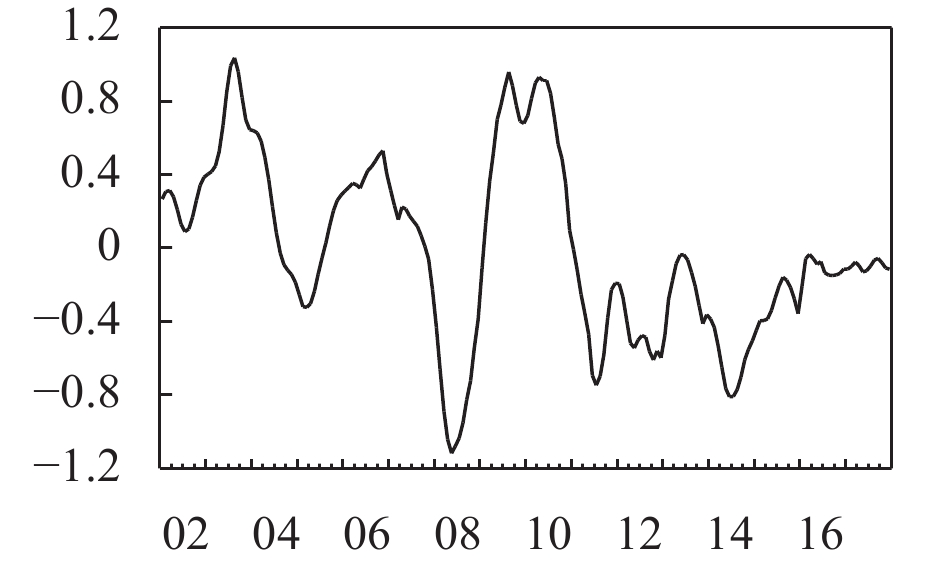图 1 中国金融形势的波动态势图 2 数量型货币政策冲击图 3 价格型货币政策冲击
(二) 金融周期与经济周期的波动特征及其关联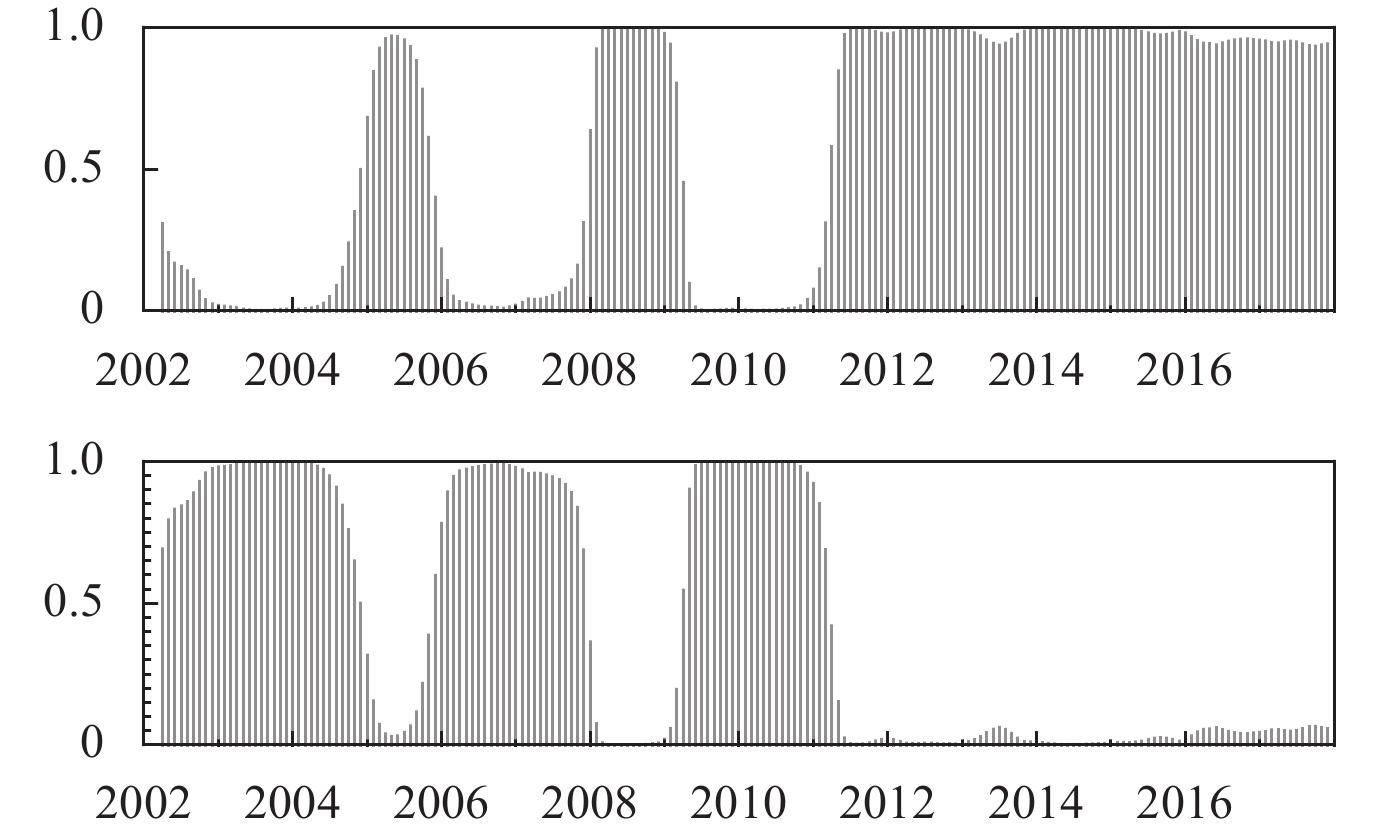图 4 金融周期双区制划分的平滑概率图 5 经济周期双区制划分的平滑概率

 金融周期 经济周期 低区制 高区制 低区制 高区制 2002.04–2004.08 2002.04–2002.11 2002.12–2008.09 2004.09–2005.07 2005.08–2007.09 2007.10–2009.01 2009.02–2011.01 2008.10–2009.09 2009.10–2012.01 2011.02–2017.12 2012.02–2017.12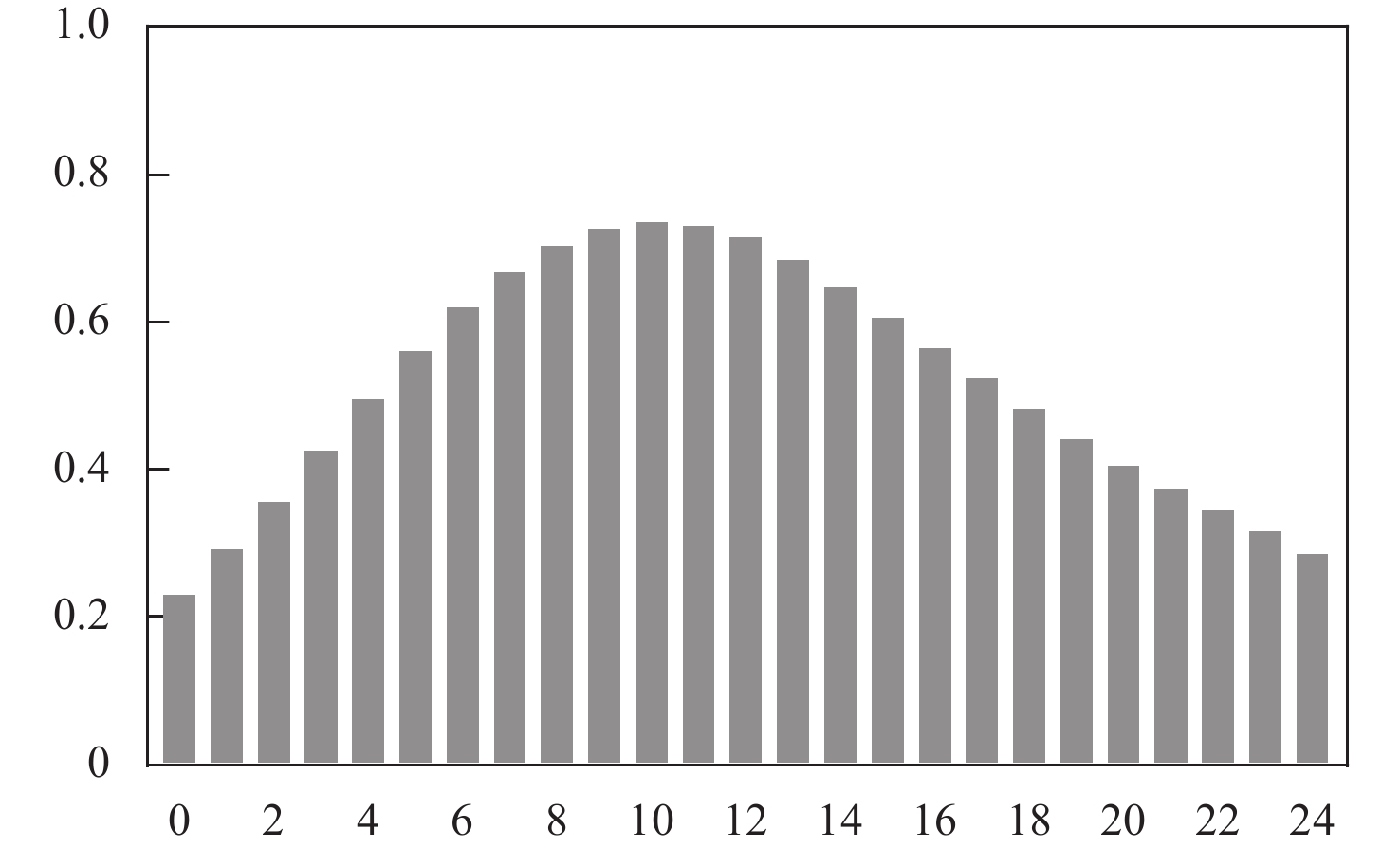图 6 金融周期与经济周期的时差相关性

 ${Y_t} = \sum\nolimits_{i = 1}^s {{\Phi _i}{Y_{t - i}}} + {\varepsilon _t}$ (3)

 ${\phi _{ij}}(H) = {{{\sigma _{jj}}^{ - 1}\sum\nolimits_{h = 0}^{H - 1} {{{({{e'}_i}{A_h}\Sigma {e_j})}^2}} } \Bigr/ {\sum\nolimits_{h = 0}^{H - 1} {({{e'}_i}{A_h}\Sigma {{A'}_h}{e_i})} }}$ (4)

 ${{\tilde \phi }_{ij}}(H) = {{{\phi _{ij}}(H)} \Bigr/ {\sum\nolimits_{j = 1}^K {{\phi _{ij}}(H)} }}$ (5)

 $TSI(H) = 100 \times {{\sum\nolimits_{i,j = 1 i \ne j}^K {{{\tilde \phi }_{ij}}(H)} } \Bigr/ {\sum\nolimits_{i,j = 1}^K {{{{\tilde \phi }}_{ij}}(H)} }} = 100 \times {{\sum\nolimits_{i,j = 1 i \ne j}^K {{{{\tilde \phi }}_{ij}}(H)} } / K}$ (6)

 $DS{I_{i \leftarrow j}}(H) = 100 \times \sum\nolimits_{j = 1,j \ne i}^K {{{{\tilde \phi }}_{ij}}} (H)$ (7)
 $DS{I_{i \to j}}(H) = 100 \times \sum\nolimits_{j = 1,j \ne i}^K {{{{\tilde \phi }}_{ji}}} (H)$ (8)

(二) 结果分析

 接收方 溢出方 用行和标准化→ 接收方 溢出方 金融周期 经济周期 金融周期 经济周期 金融周期 0.997 31 0.004 10 金融周期 0.995 91 0.004 09 经济周期 0.308 28 0.698 26 经济周期 0.306 28 0.693 72 总溢出效应 15.52% 从金融周期到经济周期的定向溢出效应 30.63% 从经济周期到金融周期的定向溢出效应 0.41%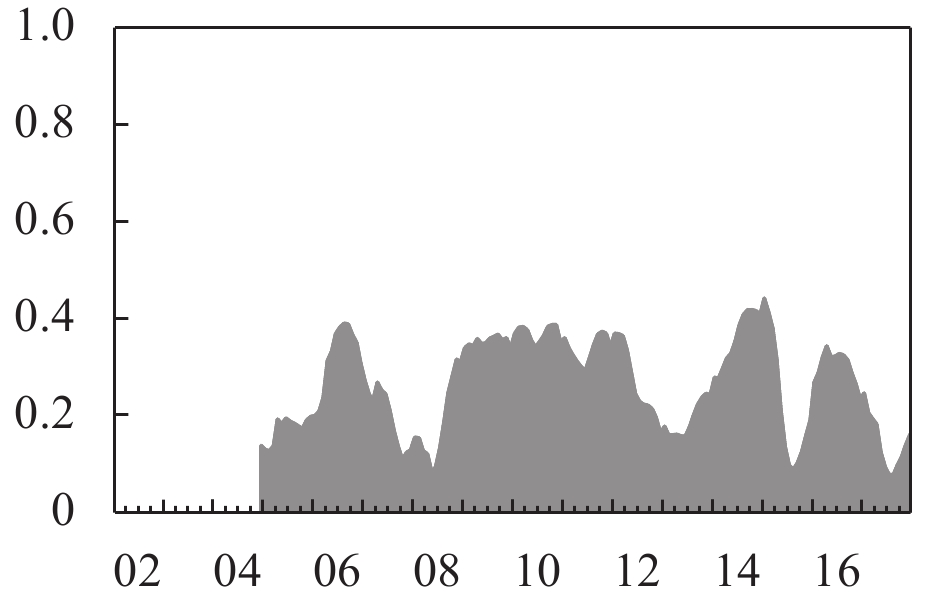图 7 总溢出指数图 8 定向溢出指数(FCI→ECI)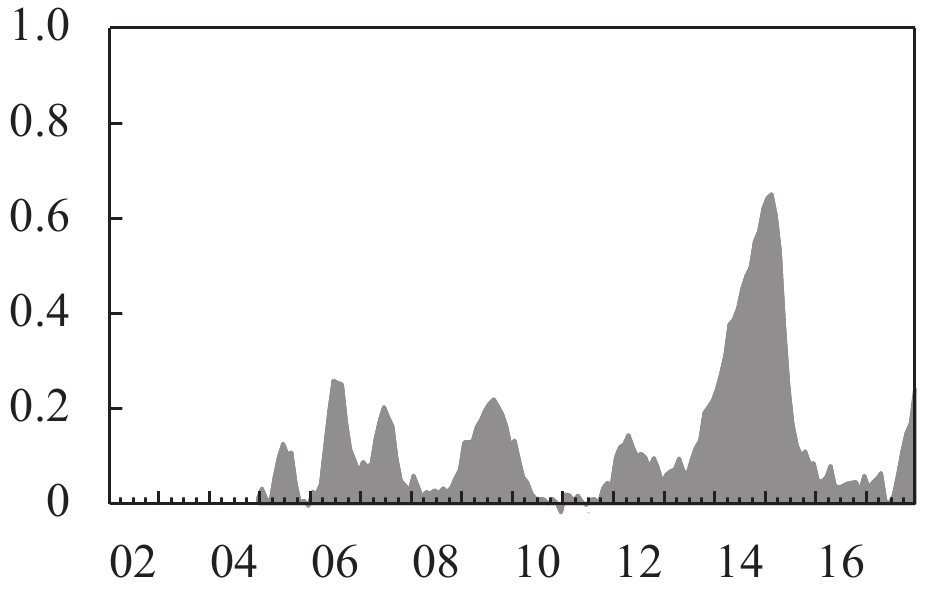图 9 定向溢出指数(ECI→FCI)

(三) 稳健性检验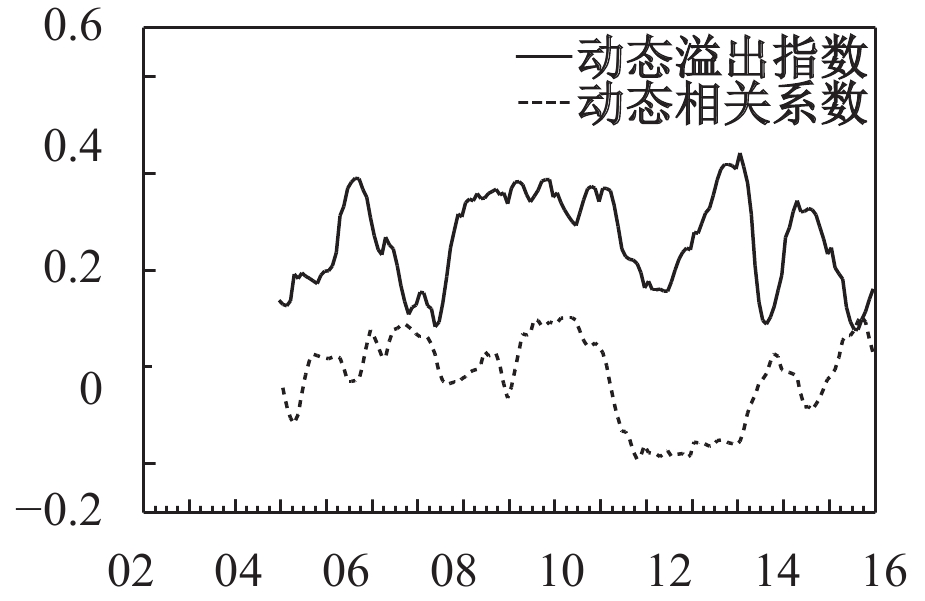图 10 总溢出指数检验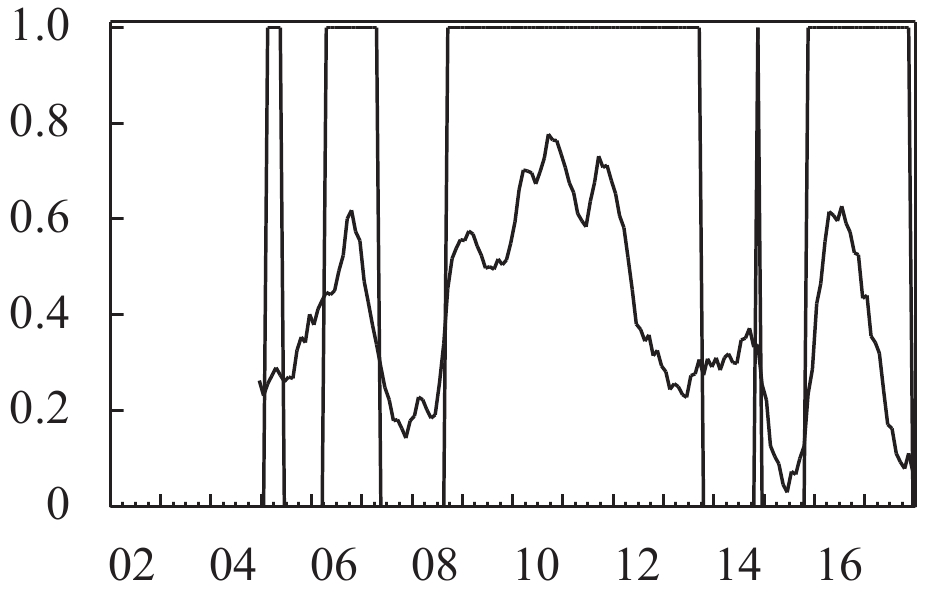图 11 定向溢出指数(FCI→ECI)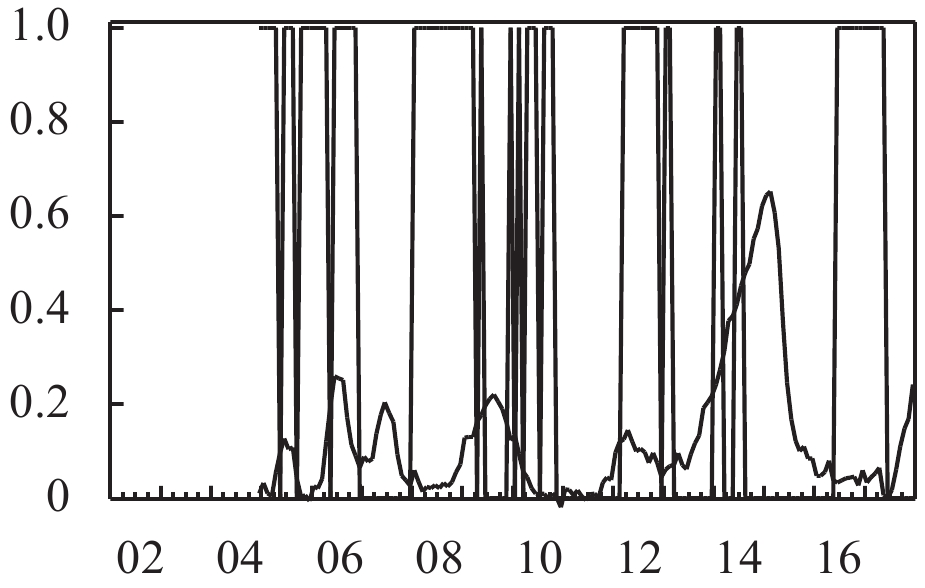图 12 定向溢出指数(ECI→FCI)

① 在货币市场和银行体系方面，货币供给和利率作为中央银行的主要货币政策工具，其变动必将间接或直接影响货币的使用成本，进而通过折现率的变化影响股市等金融子市场的变动，而社会融资规模作为能够全面反映金融对实体经济的资金支持以及金融与经济关系的总量指标，不仅包含传统金融机构贷款，更反映了近年来在金融市场化背景下影子银行的变化信息，其主要通过改变金融市场的融资条件影响企业和居民的经济行为，进而对经济和金融市场产生影响；在外汇市场方面，开放经济条件下有效汇率作为一种以对外贸易比重为权数的加权平均汇率，不仅仅是一国货币与其他国家货币联系的桥梁，更是沟通两国间物价的重要纽带，其变化必将通过引起贸易收支、资本流动和诸如股价等资产价格的变化影响一国的经济和金融形势；在股票市场和房地产市场方面，随着经济的发展，居民资产构成中除外汇资产和货币资产外，越来越多地涵盖股票和房地产等其他资产，而股价指数的变化不仅关系到公司的资本成本，而且也通过财富效应等多种渠道影响着家庭的财务和消费状况，因而是重要的金融指标之一；考虑房地产价格的主要原因在于房地产投资不仅成为居民投资组合中的重要组成部分，房价的快速上升在推动经济发展的同时也大大加剧了金融风险，因而也是度量金融市场状况不得不考虑的因素。

② 宏观一致合成指数由中国经济景气监测中心（CEMAC）编制并发布，包含工业生产、就业、社会收入（国家税收、企业利润、居民收入）、社会需求（投资、消费、外贸）四方面的信息，既能如实刻画国民经济运行情况，又因其数据频率与金融形势指数同为月度，从而能够有效规避数据频率转换所产生的与实际不符合或遗漏重要信息的风险。

③ 马尔科夫区制转移模型是探究经济周期各阶段非线性转换以及其他经济时间序列非线性特征的经典方法，篇幅所限本文不再对此模型加以赘述。

④ 为更加准确地判断本文构建的FCI的平均周期，同时避免手动挑选峰谷可能产生的误差，本文采用双区制马尔科夫区制转移模型，对金融形势指数的一阶差分序列进行区制识别，作为判断金融周期收缩阶段和扩张阶段的依据，并基于模型的估计结果根据“峰—峰”法对我国金融形势的运行态势进行周期划分。结果显示，中国自2002年以来共经历了6轮完整的金融周期。其中，周期长度最短为17个月，最长为58个月，平均为32个月，即金融形势大致表现出3年左右的周期性变动规律，因此本文选择以36期为滚动时窗考察金融周期与经济周期之间的交互影响作用。但为了突出重点和节省篇幅，文中省略了该部分的计算过程和相关结果，感兴趣的读者可向作者索取。

⑤ 模型细节参见Engle（2002），相关检验与模型估计结果受篇幅所限未在正文中列示，感兴趣的读者可向作者索取。

  曹永琴, 李泽祥. 中国金融经济周期与真实经济周期的动态关联研究[J].统计研究,2009(5).  岑丽君, 黄新克. 中国金融周期与实体经济周期关联性研究[J].商业研究,2016(4).  陈守东, 孙彦林, 刘洋. 中国金融周期与景气循环研究[J].数量经济研究,2016(1).  陈雨露. 重建宏观经济学的" 金融支柱”[J].国际金融研究,2015(6).  陈雨露, 马勇, 阮卓阳. 金融周期和金融波动如何影响经济增长与金融稳定?[J].金融研究,2016(2).  邓创, 石柱鲜. 泰勒规则与我国货币政策反应函数——基于潜在产出、自然利率与均衡汇率的研究[J].当代财经,2011(1).  邓创, 徐曼. 中国的金融周期波动及其宏观经济效应的时变特征研究[J].数量经济技术经济研究,2014(9).  封思贤, 蒋伏心, 谢启超, 等. 金融状况指数预测通胀趋势的机理与实证——基于中国1999–2011年月度数据的分析[J].中国工业经济,2012(4).  何德旭, 张捷. 经济周期与金融危机: 金融加速器理论的现实解释[J].财经问题研究,2009(10).  李成, 王彬, 马文涛. 我国金融形势指数的构建及其与宏观经济的关联性研究[J].财贸经济,2010(3).  李扬. " 金融服务实体经济”辨[J].中国经济报告,2017(6).  刘明志. 货币供应量和利率作为货币政策中介目标的适用性[J].金融研究,2006(1).  陆军, 梁静瑜. 中国金融状况指数的构建[J].世界经济,2007(4).  陆岷峰. 金融支持我国实体经济发展的有效性分析[J].财经科学,2013(6).  马勇, 冯心悦, 田拓. 金融周期与经济周期——基于中国的实证研究[J].国际金融研究,2016(10).  马勇, 张靖岚, 陈雨露. 金融周期与货币政策[J].金融研究,2017(3).  马勇, 陈雨露. 金融杠杆、杠杆波动与经济增长[J].经济研究,2017(6).  尚玉皇, 郑挺国. 中国金融形势指数混频测度及其预警行为研究[J].金融研究,2018(3).  宋玉华, 徐前春. 世界经济周期理论的文献述评[J].世界经济,2004(6).  谭小芬. 美联储量化宽松货币政策的退出及其对中国的影响[J].国际金融研究,2010(2).  王国刚. 中国金融体系改革之路[J].金融市场研究,2014(9).  王树同, 刘明学, 栾雪剑. 美联储" 量化宽松”货币政策的原因、影响与启示[J].国际金融研究,2009(11).  王维国, 王霄凌, 关大宇. 中国金融条件指数的设计与应用研究[J].数量经济技术经济研究,2011(12).  文红星. 经济金融化与经济不稳定性关系研究[J].当代经济研究,2016(7).  肖强, 司颖华. 我国FCI的构建及对宏观经济变量影响的非对称性[J].金融研究,2015(8).  徐国祥, 郑雯. 中国金融状况指数的构建及预测能力研究[J].统计研究,2013(8).  张成思, 张步昙. 再论金融与实体经济: 经济金融化视角[J].经济学动态,2015(6).  张慕濒, 诸葛恒中. 全球化背景下中国经济的金融化: 涵义与实证检验[J].世界经济与政治论坛,2013(1).  张晓晶, 王宇. 金融周期与创新宏观调控新维度[J].经济学动态,2016(7).  张晓朴, 朱太辉. 金融体系与实体经济关系的反思[J].国际金融研究,2014(3).  Bernanke B S, Gertler M, Gilchrist S. The financial accelerator in a quantitative business cycle framework[J].Handbook of Macroeconomics,1999,1:1341–1393.  Borio C. The financial cycle and macroeconomics: What have we learnt?[J]. Journal of Banking & Finance,2014,45:182–198.  Brave S A, Butters R. Monitoring financial stability: A financial conditions index approach[J]. Economic Perspectives,2011,35(1):22–43.  Christiano L, Motto R, Rostagno M. Financial factors in economic fluctuations[R]. ECB Working Paper No.1192, 2010.  Claessens S, Kose M A, Terrones M E. How do business and financial cycles interact?[J].Journal of International Economics,2012,87(1):178–190.  Da Silva G F. The impact of financial system development on business cycles volatility: Cross-country evidence[J].Journal of Macroeconomics,2002,24(2):233–253.  Diebold F X, Yilmaz K. Better to give than to receive: Predictive directional measurement of volatility spillovers[J].International Journal of Forecasting,2012,28(1):57–66.  Engle R. Dynamic conditional correlation: A simple class of multivariate generalized autoregressive conditional heteroskedasticity models[J]. Journal of Business & Economic Statistics,2002,20(3):339–350.  Fisher I. The debt-deflation theory of great depressions[J].Econometrica,1933,1(4):337–357.  Goodhart C, Hofmann B. Asset prices, financial conditions, and the transmission of monetary policy[A]. Conference on asset prices, exchange rates, and monetary policy[C]. Stanford: Stanford University, 2001.  Hamilton J D. A new approach to the economic analysis of nonstationary time series and the business cycle[J].Econometrica,1989,57(2):357–384.  Iacoviello M. House prices, borrowing constraints, and monetary policy in the business cycle[J].The American Economic Review,2005,95(3):739–764.  Meh C A, Moran K. The role of bank capital in the propagation of shocks[J].Journal of Economic Dynamics and Control,2010,34(3):555–576.  Menden C, Proaño C R. Dissecting the financial cycle with dynamic factor models[J].Quantitative Finance,2017,17(12):1965–1994.  Mimir Y. Financial intermediaries, credit shocks and business cycles[J].Oxford Bulletin of Economics and Statistics,2016,78(1):42–74.
The Interaction between Financial Cycle and Business Cycle in China: An Empirical Analysis Based on the Dynamic Spillover Index
Deng Chuang1,2, Xu Man2
1.Center for Quantitative Economics，Jilin University，Jilin Changchun 130012，China;